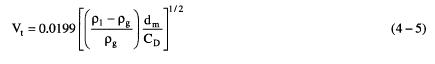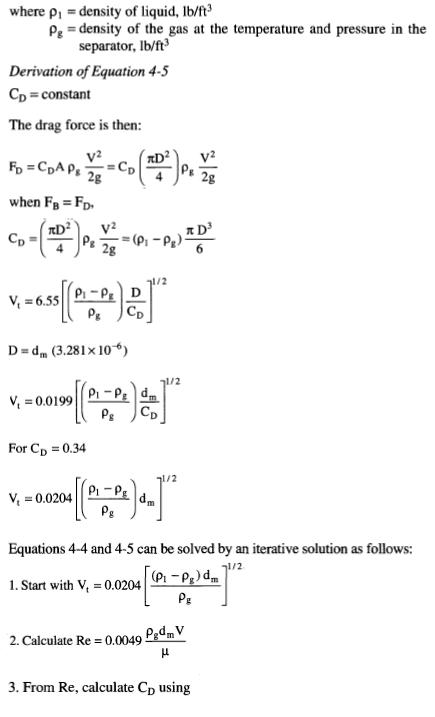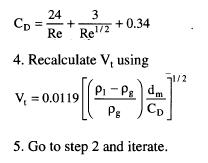﻿ Two Phase Separator Settling | Oil & Gas Process Engineering

## Two Phase Separator Settling

In the gravity settling section the liquid drops will settle at a velocity determined by equating the gravity force on the drop with the drag force caused by its motion relative to the gas continuous phase.

The drag force is determined from the equation: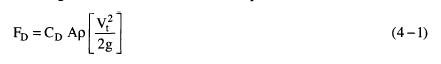where FD = drag force, Ib
CD = drag coefficient
A = cross-sectional area of the droplet, ft2
p = density of the continuous phase, lb/ft3
Vt = terminal settling velocity of the droplet, ft/s
g = gravitational constant, 32.2 ft/s2

If the flow around the drop were laminar, then Stokes’ Law would govern and: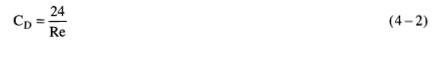where Re = Reynolds number
It can be shown that in such a gas the droplet settling velocity would be given by:Derivation of Equation 3
For low Reynolds number flows, i.e., Re < 1The buoyant force on a sphere from Archimedes’ principles is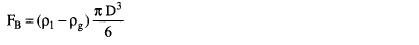When the drag force is equal to the buoyancy force, the droplet’s acceleration is zero so that it moves at a constant velocity. This is the terminal velocity.Unfortunately, for production facility design it can be shown that Stokes’ Law does not govern, and the following more complete formula for drag coefficient must be used: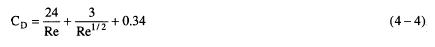Equating drag and buoyant forces, the terminal settling velocity is given by: